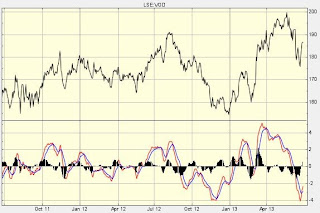## Pages

### The RSI and MACD Indicators

Moving averages (MAs) can be useful in identifying some points of support and resistance. In addition, certain crossovers between MAs of different time lengths can be used. One example of these is the 'Golden Cross' whereby a short-term MA crosses over a longer-term MA, indicating a swift upward movement. However, this alone is not particularly useful. Instead, a range of technical indicators can be used. Over 100 varieties of technical indicators exist and each of them can measure different parts of share movement. Some are compounds (such as Ichimoku charts), whereas others are standalone. Two key, useful, easy to use and widely accepted types include the Relative Strength Index and MACD indicators

Relative Strength Index (RSI)RSI for BT shares
The Relative Strength Index is not an overlay, but a separate numerical graph that can be plotted beside the share price chart. This is shown below the share price chart for BT. The RSI is plotted between 0 and 100 and fluctuates within these bands, but usually within the 30 and 70 bands. As a starting point, the 50 level represents neutral. The 30 band represents the oversold level whereas the 70 band represents the overbought level. In simple terms, when the RSI hits or falls below 30, it can be used to signal that the share is oversold and due to bounce upwards. Conversely, when the RSI hits or exceeds 70, it can represent the share as being overbought and due to pull downwards in the short-term. It is important to recognise that the RSI is a short-term indicator and should not be used independently. Nonetheless, it can be used to identify when bounces are likely to occur.

Moving Average Convergence Divergence (MACD)MACD for Vodafone shares
The MACD is an indicator that measures momentum for a particular stock and it is comprised of moving averages (thus it is a lagging indicator). In this instance you can see two lines beneath the Vodafone price chart. When the lines are below zero then the momentum for the stock is downwards and vice versa. The line to focus upon is the red "signal" line. When the signal line crosses under the blue line, it is the same as a short-term MA moving under a longer-term MA hence a sell signal is derived. Once again, if the red signal line crosses over the blue line then it is an indication that momentum is firmly upwards. The MACD is less useful when the share price is volatile, but when used in combination with the RSI, it can generate interesting readings. For Vodafone, a sell signal was generated in October 2012. The share price fell from 175p towards 157p following this signal. A buy signal was generated in December 2012 at 157p and the shares then rallied to 172p in late January. However, during this period is an example of why the MACD should not be used independently. At 157p the MACD crossed upwards indicating a buy signal, but the MACD actually generated a sell signal at 156.6p roughly one month later which represents a loss on the original investment.